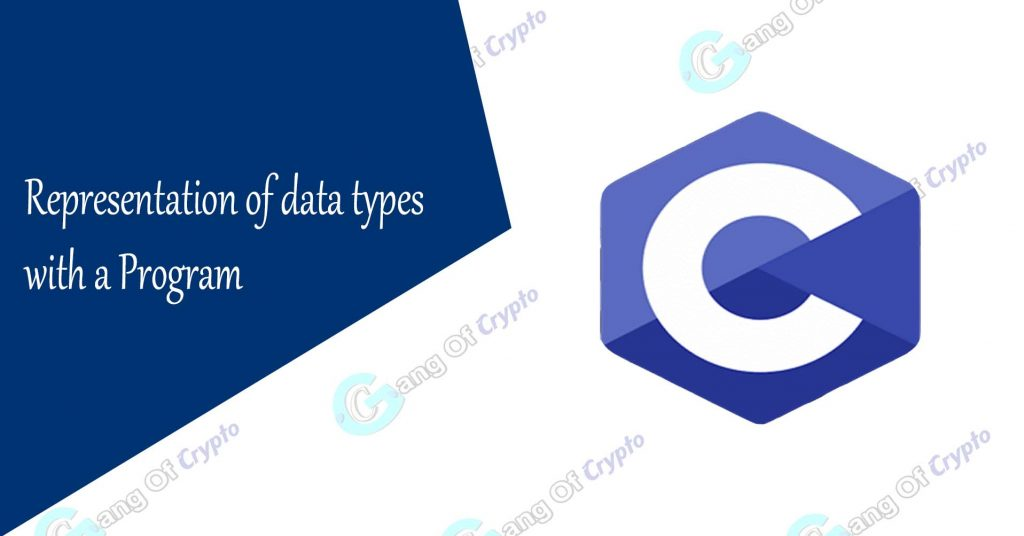# Representation of Data types in C

### Data types in C Language## How Data types are represented in C ?

Each of the primary data type is represented uniquely in C Language. This actually used to print the value of the data type in programs. To access a specific data type we need to represent it in C.

1. Integer type (int)                           –      %d
2. Floating type(float)                       –      %f
3. Character type(char)                    –      %c
4. Double type(double)                      –      %e   (or) %E
5. Long double                                     –      %lf

## Program using different data types

What we do ?

Print each kind of data type using different representations in a single C Program.

``````//Program to print Different Data Types
#include<stdio.h>
void main(){
int i;
char c;
float f;
double d;
i=2;
c='M';
f=2.35;
d=123456;
//Printing Values
printf("\n Integer is %d",i);
printf("\n Character is %c",c);
printf("\n Floar is %f",f);
printf("\n Double  is %d",d);

} ``````
OUTPUT:

integer is 2
character is M
Float is 2.35
double is 123456